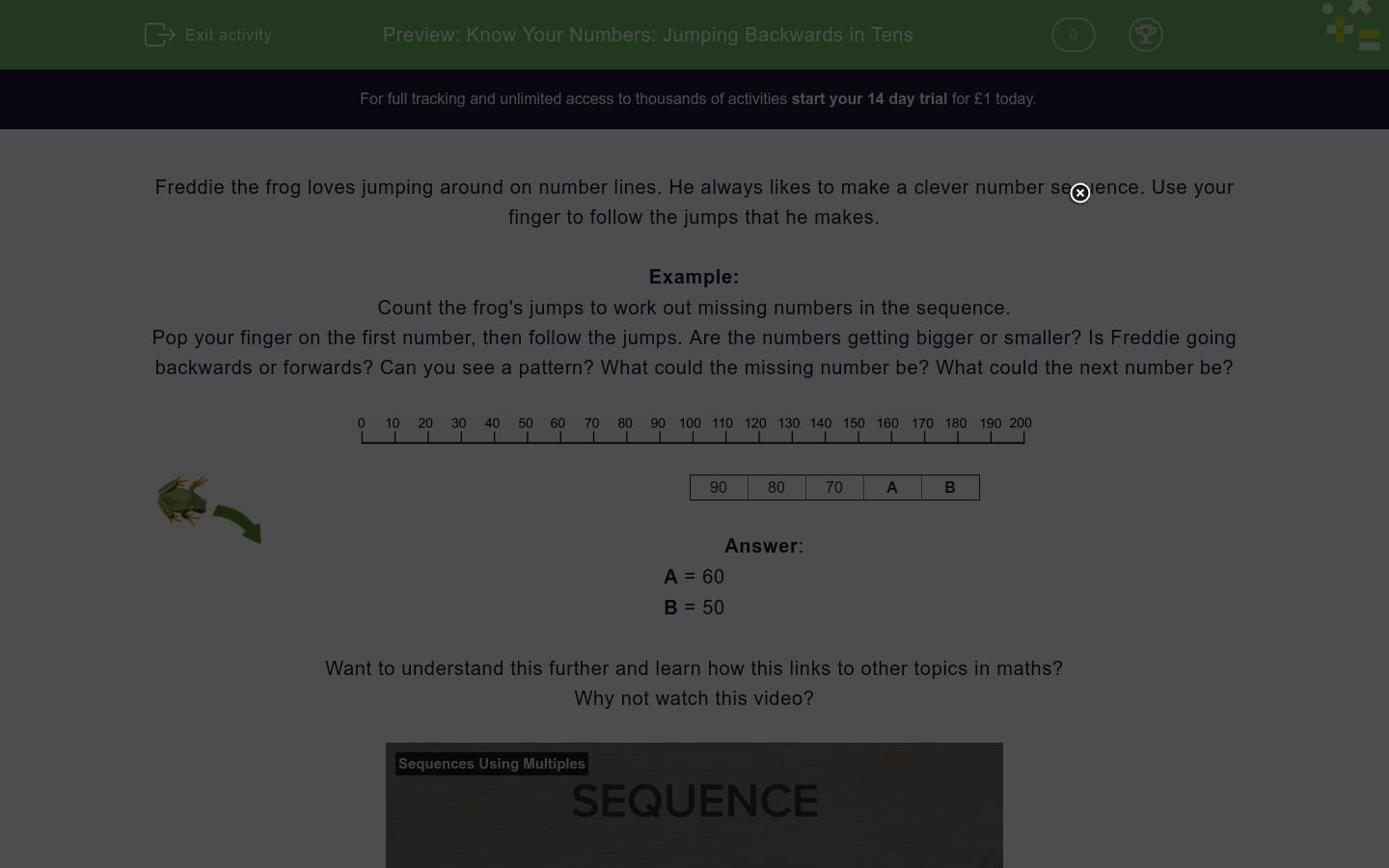# Know Your Numbers: Jumping Backwards in Tens

In this worksheet, students count backwards in jumps of ten from three- and two-digit figures to work out the next numbers in a given sequence.Key stage:  KS 2

Curriculum topic:   Number: Number and Place Value

Curriculum subtopic:   Count in Multiples (4, 8, 50 and 100)

Difficulty level:### QUESTION 1 of 10

Freddie the frog loves jumping around on number lines. He always likes to make a clever number sequence. Use your finger to follow the jumps that he makes.

Example:

Count the frog's jumps to work out missing numbers in the sequence.

Pop your finger on the first number, then follow the jumps. Are the numbers getting bigger or smaller? Is Freddie going backwards or forwards? Can you see a pattern? What could the missing number be? What could the next number be?90 80 70 A B

A = 60

B = 50

Want to understand this further and learn how this links to other topics in maths?
Why not watch this video?

Count the frog's jumps to work out missing numbers in the sequence.110 100 90 80 a b
a=70 and b=60

a=70 and b=50

a=60 and b=70

a=80 and b=60

Count the frog's jumps to work out missing numbers in the sequence.90 80 70 60 a b
a=60 and b=50

a=50 and b=40

a=70 and b=50

a=70 and b=60

Count the frog's jumps to work out missing numbers in the sequence.100 90 80 70 a b
a=70 and b=60

a=70 and b=50

a=50 and b=40

a=60 and b=50

Count the frog's jumps to work out missing numbers in the sequence.70 60 50 40 a b
a=60 and b=50

a=30 and b=20

a=20 and b=30

a=40 and b=30

Count the frog's jumps to work out missing numbers in the sequence.80 70 60 50 a b
a=30 and b=40

a=40 and b=30

a=50 and b=40

a=60 and b=50

Count the frog's jumps to work out missing numbers in the sequence.60 50 40 30 a b
a=20 and b=10

a=10 and b=20

a=40 and b=30

a=30 and b=40

Count the frog's jumps to work out missing numbers in the sequence.140 130 120 110 a b
a=90 and b=80

a=110 and b=100

a=100 and b=90

a=90 and b=100

Count the frog's jumps to work out missing numbers in the sequence.150 140 130 120 a b
a=100 and b=90

a=110 and b=100

a=90 and b=100

a=100 and b=110

Count the frog's jumps to work out missing numbers in the sequence.120 110 100 90 a b
a=70 and b=80

a=60 and b=50

a=80 and b=70

a=90 and b=80

Count the frog's jumps to work out missing numbers in the sequence.130 120 110 100 a b
a=100 and b=110

a=90 and b=80

a=80 and b=90

a=100 and b=90

• Question 1

Count the frog's jumps to work out missing numbers in the sequence.110 100 90 80 a b
a=70 and b=60
EDDIE SAYS
110, 100, 90, 80, 70, 60 is the sequence.
Freddie is jumping backwards 10 places each time.
• Question 2

Count the frog's jumps to work out missing numbers in the sequence.90 80 70 60 a b
a=50 and b=40
EDDIE SAYS
90, 80, 70, 60, 50, 40 is the sequence.
Freddie is jumping backwards 10 places each time.
• Question 3

Count the frog's jumps to work out missing numbers in the sequence.100 90 80 70 a b
a=60 and b=50
EDDIE SAYS
100, 90, 80, 70, 60, 50 is the sequence.
Freddie is jumping backwards 10 places each time.
• Question 4

Count the frog's jumps to work out missing numbers in the sequence.70 60 50 40 a b
a=30 and b=20
EDDIE SAYS
70, 60, 50, 40, 30, 20 is the sequence.
Freddie is jumping backwards 10 places each time.
• Question 5

Count the frog's jumps to work out missing numbers in the sequence.80 70 60 50 a b
a=40 and b=30
EDDIE SAYS
80, 70, 60, 50, 40, 30 is the sequence.
Freddie is jumping backwards 10 places each time.
• Question 6

Count the frog's jumps to work out missing numbers in the sequence.60 50 40 30 a b
a=20 and b=10
EDDIE SAYS
60, 50, 40, 30, 20, 10 is the sequence.
Freddie is jumping backwards 10 places each time.
• Question 7

Count the frog's jumps to work out missing numbers in the sequence.140 130 120 110 a b
a=100 and b=90
EDDIE SAYS
140, 130, 120, 110, 100, 90 is the sequence.
Freddie is jumping backwards 10 places each time.
• Question 8

Count the frog's jumps to work out missing numbers in the sequence.150 140 130 120 a b
a=110 and b=100
EDDIE SAYS
150, 140, 130, 120, 110, 100 is the sequence.
Freddie is jumping backwards 10 places each time.
• Question 9

Count the frog's jumps to work out missing numbers in the sequence.120 110 100 90 a b
a=80 and b=70
EDDIE SAYS
120, 110, 100, 90, 80, 70 is the sequence.
Freddie is jumping backwards 10 places each time.
• Question 10

Count the frog's jumps to work out missing numbers in the sequence.130 120 110 100 a b
a=90 and b=80
EDDIE SAYS
130, 120, 110, 100, 90, 80 is the sequence.
Freddie is jumping backwards 10 places each time.
---- OR ----

Sign up for a £1 trial so you can track and measure your child's progress on this activity.

### What is EdPlace?

We're your National Curriculum aligned online education content provider helping each child succeed in English, maths and science from year 1 to GCSE. With an EdPlace account you’ll be able to track and measure progress, helping each child achieve their best. We build confidence and attainment by personalising each child’s learning at a level that suits them.

Get started